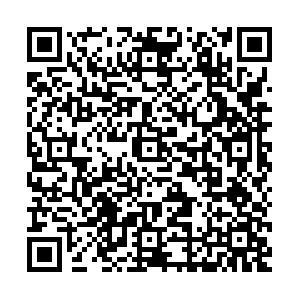# Search for QCD critical point by transverse velocity dependence of anti-deuteron to deuteron ratio

• We propose the transverse velocity ($\beta_T$) dependence of the anti-deuteron to deuteron ratio as a new observable to search for the QCD critical point in heavy-ion collisions. The QCD critical point can attract the system evolution trajectory in the QCD phase diagram, which is known as the focusing effect. To quantify this effect, we employ the thermal and hadronic transport model to simulate the dynamical particle emission along a hypothetical focusing trajectory near the critical point. We found that the focusing effect can lead to anomalous $\beta_T$dependence on $\bar{p}/p$, $\bar{d}/d$and $^3\overline{\rm{He}}/^3{\rm{He}}$ratios. We examined the $\beta_T$dependence of $\bar{p}/p$and $\bar{d}/d$ratios of central Au+Au collisions at $\sqrt {s_{\rm{NN}}} =$7.7 to 200 GeV measured by the STAR experiment at RHIC. Surprisingly, we only observe a negative slope in $\beta_T$dependence of $\bar{d}/d$ratio at $\sqrt {s_{\rm{NN}}} =$19.6 GeV, which indicates the trajectory evolution has passed through the critical region. In the future, we could constrain the location of the critical point and/or width of the critical region by conducting precise measurements on the $\beta_T$dependence of the $\bar{d}/d$ratio at different energies and rapidity.
••Get Citation
Ning Yu, Dingwei Zhang and Xiaofeng Luo. Search for QCD critical point by transverse velocity dependence of anti-deuteron to deuteron ratio[J]. Chinese Physics C, 2020, 44(1): 014002. doi: 10.1088/1674-1137/44/1/014002
Ning Yu, Dingwei Zhang and Xiaofeng Luo. Search for QCD critical point by transverse velocity dependence of anti-deuteron to deuteron ratio[J]. Chinese Physics C, 2020, 44(1): 014002.Milestone
Article Metric

Article Views(1056)
Cited by(0)
Policy on re-use
To reuse of subscription content published by CPC, the users need to request permission from CPC, unless the content was published under an Open Access license which automatically permits that type of reuse.
###### 通讯作者: 陈斌, bchen63@163.com
• 1.

沈阳化工大学材料科学与工程学院 沈阳 110142

Title:
Email:

## Search for QCD critical point by transverse velocity dependence of anti-deuteron to deuteron ratio

###### Corresponding author: Xiaofeng Luo, xfluo@mail.ccnu.edu.cn
• 1. School of Physics & Electronic Engineering, Xinyang Normal University, Xinyang 464000, China
• 2. Key Laboratory of Quark & Lepton Physics (MOE) and Institute of Particle Physics, Central China Normal University, Wuhan 430079, China

Abstract: We propose the transverse velocity ($\beta_T$) dependence of the anti-deuteron to deuteron ratio as a new observable to search for the QCD critical point in heavy-ion collisions. The QCD critical point can attract the system evolution trajectory in the QCD phase diagram, which is known as the focusing effect. To quantify this effect, we employ the thermal and hadronic transport model to simulate the dynamical particle emission along a hypothetical focusing trajectory near the critical point. We found that the focusing effect can lead to anomalous $\beta_T$dependence on $\bar{p}/p$, $\bar{d}/d$and $^3\overline{\rm{He}}/^3{\rm{He}}$ratios. We examined the $\beta_T$dependence of $\bar{p}/p$and $\bar{d}/d$ratios of central Au+Au collisions at $\sqrt {s_{\rm{NN}}} =$7.7 to 200 GeV measured by the STAR experiment at RHIC. Surprisingly, we only observe a negative slope in $\beta_T$dependence of $\bar{d}/d$ratio at $\sqrt {s_{\rm{NN}}} =$19.6 GeV, which indicates the trajectory evolution has passed through the critical region. In the future, we could constrain the location of the critical point and/or width of the critical region by conducting precise measurements on the $\beta_T$dependence of the $\bar{d}/d$ratio at different energies and rapidity.

### HTML关注分享DownLoad:  Full-Size Img  PowerPoint